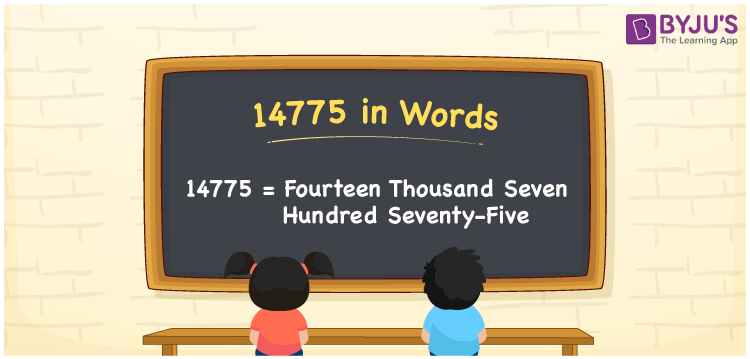# 14775 in Words

In English words, the number 14775 is written as Fourteen thousand seven hundred seventy-five. The number 14775 is a cardinal number. Also, 14775 is a natural number that lies between 14774 and 14776. In this article, we are going to learn the method of writing the number 14775 in words using the place value system in detail.

 14775 in Words: Fourteen Thousand Seven Hundred Seventy-five. Fourteen Thousand Seven Hundred Seventy-five in Numerical Form: 14775.

## 14775 in English Words## How to Write 14775 in Words?

14775 has five digits and its place values are given below:

 Ten-thousands Thousands Hundreds Tens Ones 1 4 7 7 5

The expanded form of 14775 is as follows:

= 1 × Ten thousand + 4 × Thousand + 7 × Hundred + 7 × Ten + 5 × One

= 1 × 10000 + 4 × 1000 + 7 × 100 + 7 × 10 + 5 × 1

= 10000 + 4000 + 700 + 70 + 5

= 14775

= Fourteen thousand seven hundred seventy-five

Hence, 14775 in words is fourteen thousand seven hundred seventy-five.

14775 in words – Fourteen thousand seven hundred seventy-five

Is 14775 an odd number? – Yes

Is 14775 an even number? – No

Is 14775 a perfect square number? – No

Is 14775 a perfect cube number? – No

Is 14775 a prime number? – No

Is 14775 a composite number? – Yes

## Frequently Asked Questions on 14775 in Words

Q1

### Write 14775 in words.

14775 in words is fourteen thousand seven hundred seventy-five.

Q2

### Simplify 14000 + 775, and express it in words.

Simplifying 14000 + 775, we get 14775. Hence, 14775 in words is fourteen thousand seven hundred seventy-five.

Q3

### Is 14775 an odd number?

Yes, 14775 is an odd number.